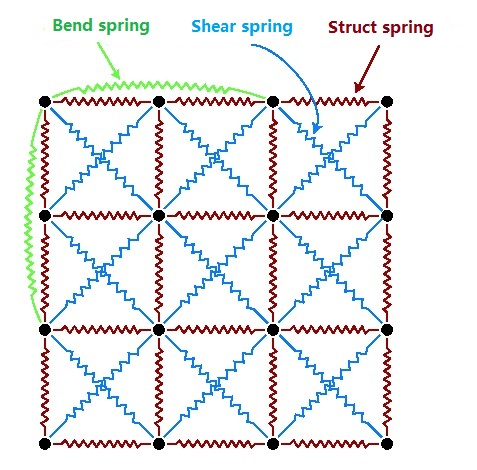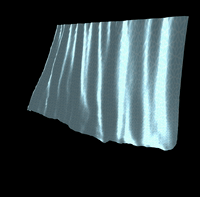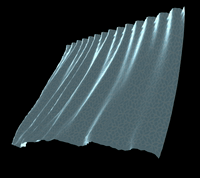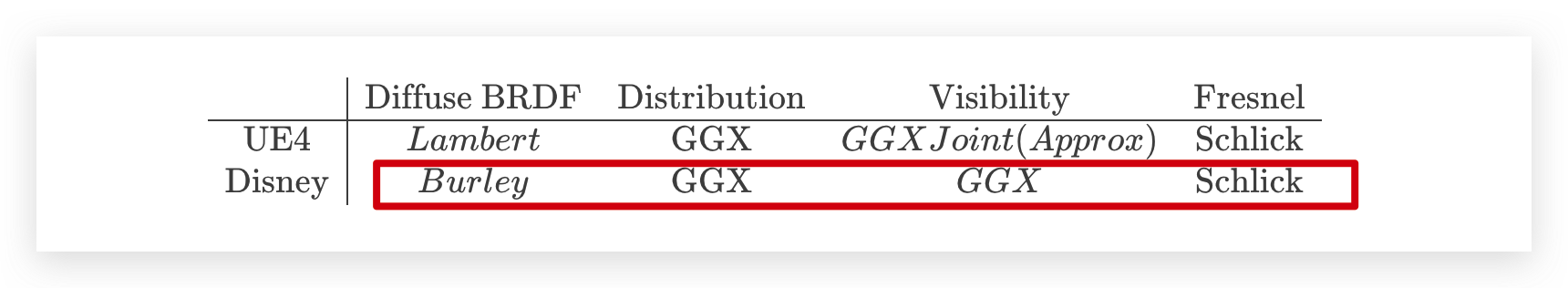## 前言demo地址：https://xiexuefeng.cc/app/we/demo/cloth-render/

## 弹簧质点系统

### 构建质点网格### 约束条件

1. 力的约束：在弹簧质点系统里面指的就是各种弹簧的构造，在网格中来说具体就是：
• 结构弹簧：即网格中相邻两点之间的连接，模拟拉力或压力；
• 剪切弹簧：即网格中对角线之间的连接，模拟剪力；
• 弯曲弹簧：即网格中间隔一点之间的连接，模拟弯矩；
2. 距离的约束：由于现实中的弹簧不可能无限拉伸或无限压缩，因此需要给定一个最长长度和最短长度，当出现要超过这个合理范围的长度时，此时弹簧表现为刚体，长度处于临界状态；其实也可以用距离约束来模拟布料的弹性，弹性大自然可以拉得更长，反之弹性小自然就拉得更短；
3. 碰撞约束：按理说为了预防布料之间的质点进行穿模，应该要加上碰撞检测进行约束……

## 质点位置计算

$\vec{x}(t + \Delta{t}) = 2\vec{x}(t) - \vec{x}(t - \Delta{t}) + \vec{a}(t)*\Delta{t}^2$

$\vec{x}(t + \Delta{t}) = \vec{x}(t) + (\vec{x}(t) - \vec{x}(t - \Delta{t})) * (1 - K_{damping}) + \vec{a}(t)*\Delta{t}^2$

## 风力场

$F(\vec{x}, t) = \begin{bmatrix} f_x(\vec{x}, t) \\[0.5em] f_y(\vec{x}, t) \\[0.5em] f_z(\vec{x}, t) \end{bmatrix}$

### 示例

$F(\vec{x}, t) = \begin{bmatrix} 0 \\[0.5em] -min(0, 0.35 * sin(0.25*t + 0.3*yz - z)) \\[0.5em] -min(0, 0.45 * sin(2*t + 0.3*xy - z)) \end{bmatrix}$$F(\vec{x}, t) = \begin{bmatrix} 0 \\[0.5em] 0 \\[0.5em] -min(0, 0.45 * sin(2*t + 0.3*xy - z)) \end{bmatrix}$### 需要注意的地方

1. 这里的系数实际上取决于每个质点的质量及弹簧力之间的相对关系；
2. 构造力场得到力应该与其原本的力（弹簧力和重力等）处于同一数量级，不然很容易会让弹簧质点系统的运动处于不收敛状态；

## BRDF